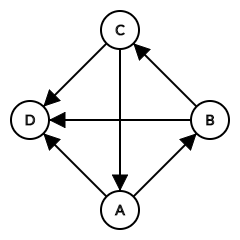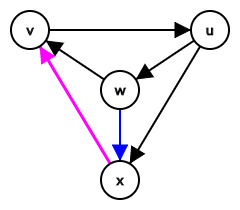### 题目描述

• 对于任意四点 $v_1, v_2, v_3, v_4$，它们在 $G$ 中导出的子图不同构于下图：### 输入格式

$n$ 行中的第 $i$ ($1 \leq i \leq n$) 行为一个长度为 $\dfrac n4$ 的 $16$ 进制串 (可以包含前导 $0$)，将这个 $16$ 进制串看成一个 $16$ 进制数，那么将其转成 $n$ 位二进制数 (可以包含前导 $0$)后，从高位起第 $j$ 位 ($1 \leq j \leq n$，记作 $G_{i, j}$) 表示是否存在 $i \to j$ 这条有向边。

### 题解

(ps: 以下内容为 2019.5.16 更新)(事实上，注意到如果 $v \to x$ 的话 $\left( v, u, w; x \right)$ 四点组会与题目条件矛盾)

### 代码

#include <bits/stdc++.h>
#define for_bitset(variable, bitset) for (variable = bitset._Find_first(); variable != N; variable = bitset._Find_next(variable))
using std::cin;
using std::cout;

typedef long long ll;
const int N = 8000;
typedef std::bitset <N> bitset;

int n, inf;
int trs;
bitset Gi[N], Go[N];
char s[N];
int pre[N];

int cnt = 0, id[N], low[N];
int stc = 0, sta[N];
int scc = 0, bel[N], size[N];
bool instack[N];

inline void down(int &x, const int y) {x > y ? x = y : 0;}

void dfs(int x) {
int y;
id[x] = low[x] = cnt++, instack[x] = true, sta[stc++] = x;
for_bitset (y, Go[x])
if (!~id[y])
dfs(y), down(low[x], low[y]);
else if (instack[y])
down(low[x], id[y]);
if (id[x] == low[x]) {
for (y = -1; y != x; )
y = sta[--stc], instack[y] = false, bel[y] = scc, ++size[scc];
++scc;
}
}

int main() {
int i, j, c, d, K1, K2, K3; ll ans = 0;
std::ios::sync_with_stdio(false), cin.tie(NULL);
std::iota(trs + 48, trs + 58, 0), std::iota(trs + 65, trs + 71, 10);
cin >> n, inf = 614 * n;
for (i = 0; i < n; ++i)
for (cin >> s, j = c = 0; c < n / 4; ++c, j += 4)
d = trs[(int)s[c]],
Go[i].set(j + 0, d & 8), Gi[j + 0].set(i, d & 8),
Go[i].set(j + 1, d & 4), Gi[j + 1].set(i, d & 4),
Go[i].set(j + 2, d & 2), Gi[j + 2].set(i, d & 2),
Go[i].set(j + 3, d & 1), Gi[j + 3].set(i, d & 1);
memset(id, -1, n << 2);
for (i = 0; i < n; ++i) if (!~id[i]) dfs(i);
assert(scc), assert(std::count(size + 1, size + scc, 1) == scc - 1);
ans += (n - *size) * (n + *size - 1ll) / 2 * (1 + inf);
for (i = 0; i < n; ++i) if (bel[i])
for (Go[i].reset(), j = 0; j < n; ++j) Gi[j].reset(i);
for (i = 0; i < n; ++i) if (!bel[i]) {
int &p = pre[i]; p = -1;
for_bitset (j, Go[i]) (~p && Gi[p].test(j)) || (p = j);
}
for (K2 = i = 0; i < n; ++i) if (!bel[i])
for (j = 0; j < n; ++j) if (!bel[j])
K2 += Gi[i].test(j) && ~pre[i] && Go[ pre[i] ].test(j);
K1 = *size * (*size - 1) / 2, K3 = K1 - K2;
printf("%lld\n", ans + K1 + K2 * 2 + K3 * 3);
return 0;
}
syntax_highlight()